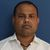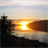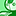# QlikView App Dev

Discussion Board for collaboration related to QlikView App Development.

cancel
Showing results for
Did you mean:Creator III

## Need help on previous Week's WTD set analysis on current year

Hi,

I am using the below set analysis for WTD calculation:

sum({\$<[Process Date]={">=\$(=WeekStart(Max([Process Date]))) <=\$(=Max([Process Date]))"}>}[Sales Posting Amount USD])

But now I have to calculate Previous Week's(But not previous Year's WTD, so if it 4th week of year, I need 3rd weeks WTD) WTD and please give me the set analysis for that.

Thanks,

Sandip

4 RepliesMVP

May be this

Sum({\$<[Process Date]={">=\$(=WeekStart(Max([Process Date]), -1)) <=\$(=Date(Max([Process Date])-7))"}>}[Sales Posting Amount USD])Master III

Hi,

may be

WeekStart(Max(Date),-1)

WeekEnd(Max(Date),-1)

Regards,

AntonioSpecialist

Hi

Create a Calendar and use set Analysis to define all metrics based on the calendar filter.

if you are in the middle of the week and you want to compare with previous week then you have to compare number of days passed in the current week with same number of days of last week in order to have an equal comparative.

Compare Current Period with Previous Period MistakeContributor III

Thanks @sunny_talwar your formula helped for me

I really appreciate it boss i was trying such calculations from yesterday 11 am

thanks thank u it helped